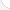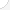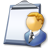Teachers-adult literacy and remedial and self-enrichment educationDescription: Adult education teachers work with people who want to update their job skills or prepare for the GED exam. They also teach writing, reading, and mathSource: Bureau of Labor Statistics, U.S. Department of Labor, Occupational Outlook Handbook, 2008-09 Edition at http://www.bls.gov/OCO/Complete Job Profile: http://www.bls.gov/oco/ocos289.htmSalary: \$28,001 to \$50,000 per yearComments:

There are 70 math topics Teachers-adult literacy and remedial and self-enrichment education need to know.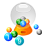Basic Math / Algebra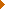FractionsDecimalsRatio and ProportionPercentCustomary MeasurementMetric MeasurementMeasurement ConversionBasic ProbabilityBasic StatisticsStatistical GraphingPowers and RootsOther Number BasesNegative NumbersScientific NotationBasic Problem Solving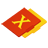First-Year AlgebraUsing FormulasLinear EquationsLinear InequalitiesOperations with PolynomialsFactoring PolynomialsRational ExpressionsCoordinate Graphing 2DLinear SystemsRadicalsQuadratic EquationsAlgebraic Representation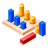GeometryBasic TerminologyAngle MeasurementCongruent TrianglesTriangle InequalitiesParallel LinesQuadrilateralsSimilarityGeometric MeanPythagorean TheoremRight Triangle TrigonometryCirclesConstructionsAreaVolumeTransformationsMake/Use 3D Drawings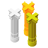Second-Year Algebra / TrigonometryFunctionsVariationImaginary NumbersPolynomial EquationsLogarithmsSequences and SeriesMatricesCoordinate Graphing 3DAdvanced ProbabilityAdvanced StatisticsConic SectionsNon-Linear SystemsTrigonometric/Circular FunctionsGraphs of Trigonometric FunctionsTrigonometric IdentitiesTrigonometric Equations/InversesOblique TrianglesPolar Coordinates/GraphsVectors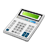Other TopicsCalculus and Higher MathBasic Calculator UseScientific Calculator UseComputer UseComputer ProgrammingGroup Problem SolvingInductive/Deductive ReasoningMath CommunicationsMathematical Modeling